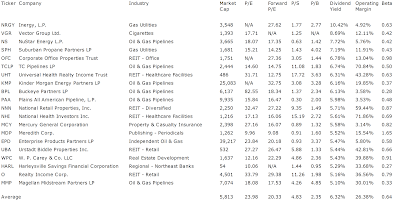## Pages

### 20 High Yield Dividend Contenders

High Yield Dividend Contenders by Dividend Yield - Stock, Capital, Investment. Here is a current sheet stocks that have raised their dividends for at least 10 years in a row but not more than 25 years. 144 stocks have achieved this of which 10 are high yields with a dividend yield of more than 5 percent.

Here are the 3 top dividend stocks by dividend yield:

Inergy (NYSE:NRGY) has a market capitalization of \$3.55 billion. The company employs 2,997 people, generates revenues of \$1,786.00 million and has a net income of \$48.70 million. The firm’s earnings before interest, taxes, depreciation and amortization (EBITDA) amounts to \$299.60 million. Because of these figures, the EBITDA margin is 16.77 percent (operating margin 7.72 percent and the net profit margin finally 2.73 percent).

Here are the price ratios of the company: The P/E ratio is not calculable, Price/Sales 1.99 and Price/Book ratio 1.09. Dividend Yield: 10.38 percent. The beta ratio is 0.63.Long-Term Stock Chart Of Inergy, L.P. (Click to enlarge)

Vector Group (NYSE:VGR) has a market capitalization of \$1.39 billion. The company employs 512 people, generates revenues of \$1,063.29 million and has a net income of \$54.08 million. The firm’s earnings before interest, taxes, depreciation and amortization (EBITDA) amounts to \$122.10 million. Because of these figures, the EBITDA margin is 11.48 percent (operating margin 10.47 percent and the net profit margin finally 5.09 percent).

Here are the price ratios of the company: The P/E ratio is 17.68, Price/Sales 1.30 and Price/Book ratio not calculable. Dividend Yield: 8.77 percent. The beta ratio is 0.42.Long-Term Stock Chart Of Vector Group Ltd. (Click to enlarge)

NuStar Energy (NYSE:NS) has a market capitalization of \$3.67 billion. The company employs 1,413 people, generates revenues of \$4,403.06 million and has a net income of \$238.97 million. The firm’s earnings before interest, taxes, depreciation and amortization (EBITDA) amounts to \$456.36 million. Because of these figures, the EBITDA margin is 10.36 percent (operating margin 6.87 percent and the net profit margin finally 5.43 percent).

The total debt representing 39.68 percent of the company’s assets and the total debt in relation to the equity amounts to 79.07 percent. Due to the financial situation, the return on equity amounts to 7.91 percent. Finally, earnings per share amounted to \$3.14 of which \$4.28 were paid in form of dividends to shareholders.

Here are the price ratios of the company: The P/E ratio is 18.07, Price/Sales 0.81 and Price/Book ratio 1.35. Dividend Yield: 7.90 percent. The beta ratio is 0.42.Long-Term Stock Chart Of NuStar Energy L.P. (Click to enlarge)

Here is the table with some fundamentals (TTM):20 High Yield Dividend Contenders (Click to enlarge)

Related stock ticker symbols:
NRGY, VGR, NS, SPH, OFC, TCLP, UHT, KMP, BPL, PAA, NNN, NHI, MCY, MDP, EPD, UBA, WPC, HARL, O, MMP

Selected Articles: